International
Tables for
Crystallography
Volume D
Physical properties of crystals
Edited by A. Authier

International Tables for Crystallography (2006). Vol. D, ch. 2.3, pp. 325-326

## Section 2.3.5. Spatial-dispersion effects

I. Gregoraa*

aInstitute of Physics, Academy of Sciences of the Czech Republic, Na Slovance 2, CZ-18221 Prague 8, Czech Republic
Correspondence e-mail: gregora@fzu.cz

### 2.3.5. Spatial-dispersion effects

| top | pdf |

For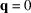, the normal modes correspond to a homogeneous phonon displacement pattern (all cells vibrate in phase). Phenomenologically, the q-dependence of Raman tensors can be understood as a kind of morphic effect due to the gradients of the displacement field. Developing the contribution of the long-wavelength jth normal mode to the susceptibility in Cartesian components of the displacement of atoms in the primitive cell and their gradients, we obtain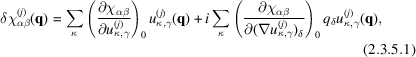where the derivatives are taken at, and we use the obvious relation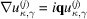.

Transforming to normal coordinates, using (2.3.3.1), we identify theintrinsic Raman tensor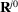of the jth normal mode, explicitly expressed via Cartesian displacements of atoms,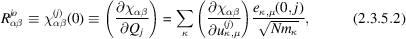and introduce the first-order q-induced atomic displacement Raman tensor coefficients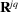: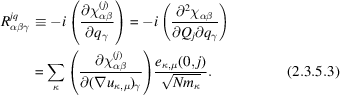Hence, to the lowest order in q, the transition susceptibility is expressed as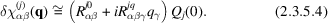In a more general case, spatial dispersion should be considered together with the electro-optic contributions due to the internal macroscopic field E and its gradients. Assuming the linear susceptibility to be modulated by the atomic displacements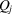and the macroscopic electric field E as well as by their gradients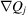and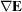, we can expand the transition susceptibility of the jth phonon mode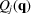to terms linear in q and formally separate the atomic displacement and electro-optic parts of the Raman tensor [see (2.3.3.15)]: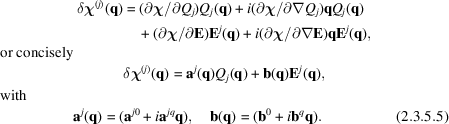Hence, setting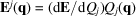, we write for the total Raman tensor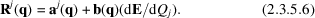The definitions of the tensors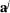and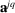correspond to (2.3.5.2)and (2.3.5.3). Analogously, the tensors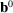and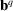are defined by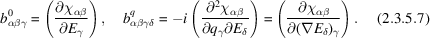The q-independent part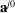of the atomic displacement Raman tensor corresponds to the standardRaman tensors, whose symmetry properties and matrix form were discussed in Section 2.3.3.3.Like, the form of the q-dependent contribution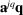also depends on the symmetry properties of the corresponding normal coordinate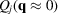. Since q (or) and E are polar vectors, the symmetry properties of theare identical to those of the coefficients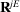of the electric-field-induced Raman tensor discussed in Section 2.3.4.2: they transform according to therepresentation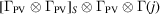. Hence, the symmetry-restricted matrix form of the q-dependent contribution to the atomic displacement Raman tensoris exactly the same as that of the corresponding electric-field-induced Raman tensor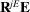. In general, these linear terms must vanish for even-parity modes in centrosymmetric crystals (where the lowest-order non-vanishing contributions to the Raman tensor are quadratic). The third-rank tensorcorresponds to the first-order susceptibility derivative b and the fourth-rank tensorto its first-order q-dependent part.

As mentioned above, the q-independent third-rank polar tensoris nonzero only in noncentrosymmetric (piezoelectric) crystals, where it contributes to Raman tensors for polar longitudinal optical (LO) phonons. The corresponding electro-optic terms in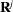, connected with the accompanying longitudinal electric field E, are given by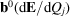. Symmetry arguments imply that the q-independent part of such terms must have the same form as the atomic displacement Raman tensor for polar LO phonons, since the corresponding normal coordinates transform as components of polar vectors.

The q-dependent part of the electro-optic contribution, the polar fourth-rank tensor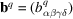, transforms as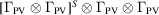and its symmetry properties are similar to those of the quadratic electro-optic tensor (however, as q and E are not interchangeable, there is no symmetry in the last two indices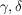). Thus, for finite q, the term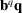has nonzero components in all crystal classes. The corresponding contribution to the Raman tensor, however, is possible only in noncentrosymmetric crystals. Again, because of the (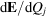) factor, the symmetry-restricted matrix form of this contribution for polar LO phonons will be equivalent to that of the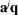term. As far as symmetry is concerned, the distinction between atomic displacement and electro-optic contributions is therefore immaterial.

The occurrence of q-dependent terms leads to polarization selection rules that are generally different from those of intrinsically () Raman-active modes. For this reason, this phenomenon is sometimes referred to as forbidden scattering. It is often observed under resonance conditions.

We recall that the terms linear in q, i.e. proportional to displacement gradients and elastic strains, are fundamental for the description of inelastic light scattering by those excitations for which the transition susceptibility identically vanishes in the limit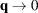regardless of lattice point symmetry. This is the case for scattering by acoustic phonons (Brillouin scattering) and also for scattering by plasma waves (plasmons in semiconductors).

We have explicitly considered only the q-dependent effects, due to gradients of phonon fields. In general, spatial dispersion may be also due to the gradients of the electric (or magnetic) field of the incident or scattered photons. The corresponding effects are often referred to as k-dependent effects. In view of the wavevector conservation condition (2.3.2.2), the three wavevectors q,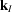and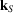are always related, which simplifies the symmetry analysis. Without going into details, we note that, microscopically, the k-dependent effects come from photon–electron interactions beyond the usual dipole approximation, i.e. from multipolar effects. The symmetry-allowed matrix form of the k-induced contributions, depending on the nature of the leading microscopic mechanism, can be obtained by standard group-theoretical techniques.

Example: As an example we give the symmetry-restricted form for the linear q-dependent contribution to the Raman tensors for Raman-inactive triply degenerate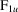modes in the m3m (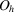) class: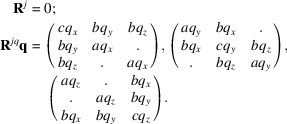The same form of the q-dependent contribution holds for Raman-active optic F2 modes in the(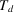) class. The conventional () intrinsic Raman tensor of F2 is nonzero, but has off-diagonal components only. Since these modes are also infrared-active, there is a concomitant splitting of LO and TO frequencies as well as a possible electro-optic contribution to the Raman tensor due to the accompanying longitudinal macroscopic field. If one chooses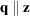, for instance, the first two matrices correspond to two degenerate TO modes and the third one to the LO mode. Combining the q-independent and q-dependent contributions, we get for each triplet of (2TOLO) F2 modes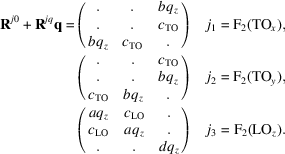The difference in the parameters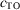and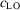is due to the electro-optic contribution to the Raman tensor for the LO mode. In the back-scattering geometry for scattering from the (001) face of the crystal, only the LOz modes can take part. Intrinsic allowed components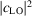are observable in the crossed (xy) polarization geometry, whereas the q-dependent terms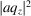appear as `forbidden' scattering in parallel (xx) or (yy) geometries.